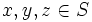# Left Bol magma with neutral element

This article defines a property that can be evaluated for a magma, and is invariant under isomorphisms of magmas.
View other such properties

## Definition

A left Bol magma with neutral element is a left Bol magma that has a neutral element. In other words, it is a magma$(S,*)$ such that:

1. For all$x,y,z \in S$, we have$\! x * (y * (x * z)) = (x * (y * x)) * z$.
2. There exists$e \in S$, we have$\! x * e = e * x = x$.

## Relation with other properties

### Stronger properties

Property Meaning Proof of implication Proof of strictness (reverse implication failure) Intermediate notions
Left Bol loop
Moufang loop

### Weaker properties

Property Meaning Proof of implication Proof of strictness (reverse implication failure) Intermediate notions
Flexible magma left Bol implies flexible
Left alternative magma left Bol implies left alternative